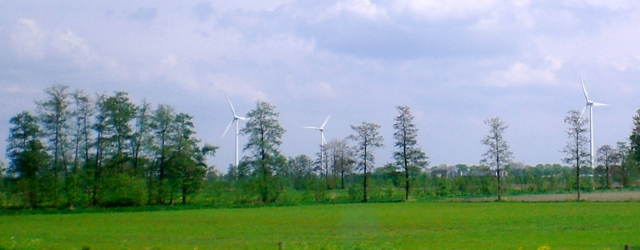# Energy: Key Terms and Definitions

Contents

## Energy

Energy is a concept that represents the amount of physical work that can be done by a certain system. For instance, a tank of gasoline has a certain amount of energy that can be released by burning it to achieve useful results, like moving a car a long distance. The concept of energy is very useful when talking about subjects as broadly different as climate change, electrical power plants, car collisions, explosives, and food diets. Energy exists in two forms, kinetic and potential.

## Kinetic

Kinetic Energy is the energy of an object in motion. This would be the ‘energy’ represented by a spinning wheel, or by a car moving down a road. An intuitive example of kinetic energy differences would be considering the idea of being hit by a soccer (football) ball or by a cannonball just fired out of a cannon. The cannonball being heavier and moving faster has much more kinetic energy than the soccer ball.

## Potential

Potential energy is stored energy. Think of a boulder on top of a big hill. It isn’t moving (relative to the ground) so it has no kinetic energy. It has potential energy because gravity is pulling down on it. If it starts rolling, the potential energy will turn into kinetic energy as gravity pulls it downwards. When the boulder stops moving, it will have less potential energy than before because some of it will have turned into kinetic energy during the rolling.

Potential energy comes in many forms.

Gravitational: The boulder on the hill is an example of gravitational potential energy.

Chemical: A tank of gasoline has a large amount of what is called chemical potential energy. By setting off a chemical reaction, we are able to release the energy stored in the gasoline.

Nuclear: Nuclear potential energy is the energy stored in the physical existence of radioactive substances. These substances ‘decay’ and give off their stored energy as heat and other forms of radiation.

Electromagnetic: Stored electricity has potential energy. This is illustrated to us every time we are ‘shocked’ by touching objects when they have a different electrical charge than us. Some common examples would be metal door handles, car doors, and chalk boards.

## Units of Energy

Joule – A joule is a unit that represents energy. One joule is the energy used to raise 1kg (kilogram, about 2.2 lbs) by 10.2 centimeters (4 inches) vertically (if you are standing on the surface of the earth). There are 4184 joules in one food calorie. Joule is symbolized as an uppercase ‘J’.

Watt – A watt is a unit that represents power. This means that it represents energy expended per second. One watt is 1 joule per second. You can think of this as raising 1kg by 10.2cm per second. A familiar unit of power is the horsepower. 1 horsepower = 746 watts. If you are interested in what a Watt or Joule is in your favourite units, check out this page. Watt is symbolized by an uppercase ‘W’.

Kilowatt – 1000 Watts as per the SI unit system, kilo means one thousand, mega means one million.  Kilowatt is symbolized as ‘kW’. Megawatt is symbolized as ‘MW’.

Kilowatt-hour – This is actually a unit of energy, not power. The meaning of kilowatt-hour is clear when we look at what each word means: 1kilowatt = 1000Watts, and 1 hour = 3600 s (seconds). 1 kilowatt-hour is thus equal to 1000W x 3600s = 3,600,000 W-s = 3,600,000 Joules. (Approx 3413 British Thermal Units.)

## Energy Costs

### Cost-per-watt

Cost-per-watt is the most common metric for comparing the costs of different forms of electrical production. The term ‘Cost-per-watt’ can mean any one of several things.

Cost-per-installed-watt: The cost of building the initial machinery and infrastructure. This would be for instance, the cost of materials, construction, and connection of any form of power plant. This does not include cost of upkeep or fuel.

Cost-per-watt (upkeep, fuel): The cost of operating the power plant once construction and hookup are completed. Basically this is a combination of fuel, upkeep, connection, and management costs.

Modified Cost-per-watt: Combine the cost of building the plant, with the total cost of its operation during its lifetime. Divide this by the total energy it produced during its lifetime. This gives you the true cost per watt of the power plant, when it’s entire (projected) lifetime is considered. This is the most fair metric for measuring the performance of power plants because it balances fuel costs against installed costs. This is what long-term planners would pay the most attention to.#### Ben Harack

I'm an aspiring omnologist who is fascinated by humanity's potential.